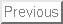: The Collatz Problem

# Curious Properties of Reiterated Processes and Beautiful Graphs made by them.

Ryohei Miyadera, Daisuke Minematsu, Taishi Inoue, Takuma Nakaoka, Koichiro Nishimura, Masakazu Naito, Yuuki Tomari, Norihiko Yamaguchi and Naoyuki Totani
Kwansei Gakuin High School and Kwansei Gakuin University, Nishinomiya City JAPAN

Abstract

In this article we are going to study reiterated processes and present beautiful graphs made by these processes. First we study well known reiterated processes such as Collatz conjecture and the digit cube sum. Collatz conjecture is an unsolved problem, but it produces beautiful graphs and we are going to present them with the computer algebra system Mathematica. The digit cube sum is an interesting problem, but its properties is easy to understand if we use Mathematica.
Next we are going to study a new reiterated process. This is the digit sum process. We define the dsf function by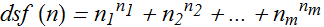,where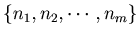is the list of the digits of an integer n. we start with any non-negative integer n, and repeatedly apply the function dsf, then we can generate a sequence of integers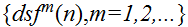. This process has very interesting properties and beautiful graphs. If you want to study this process throughly, you need the calculation by computer languages such as C and the computer algebra system Mathematica. By the calculating with Mathematica we have discovered that this process has 6 loops and 2 fixed points, and have proved this fact by two different computer programs.
One is a Mathematica program and the other is a C++ program.
These two programs are made by two completely different algorithms, and hence there is a very small chance of error.
These loops of digit sum process are registered as , , ,  and  by the On-Line Encyclopedia of Integer Sequences maintained by AT& T Labs-Research.
Next we are going to study dsfK process. We define dsfK(n) = Mod(dsf(n), K), where Mod(dsf(n), K) is the residue of dsf(n) divided by K.
Next we are going to study another new reiterated process that we call a digit factorial sum process. we define dfsf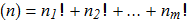whereis the list of the digits of an integer n.
This process has interesting properties and beautiful graphs, too.: The Collatz Problem